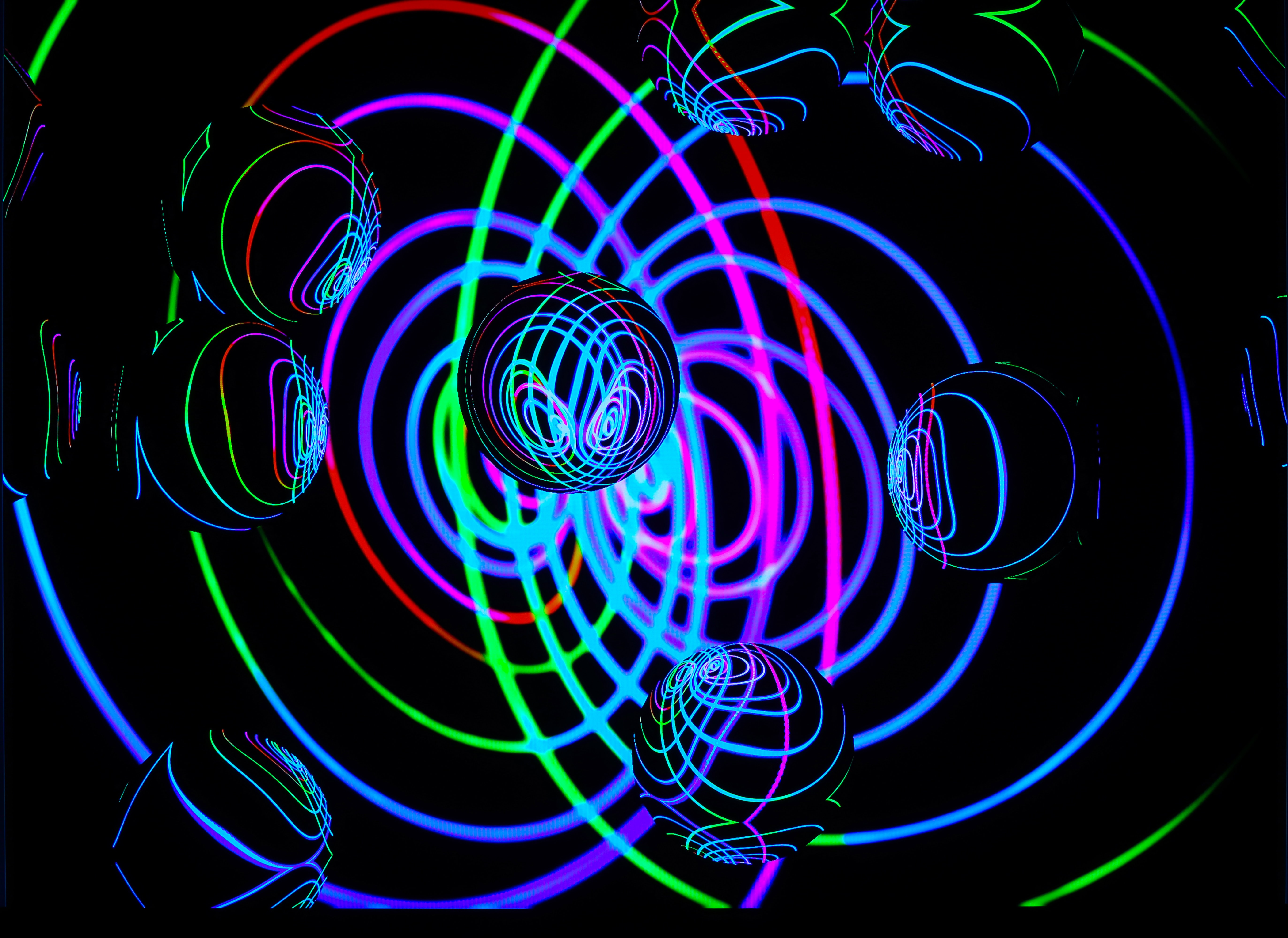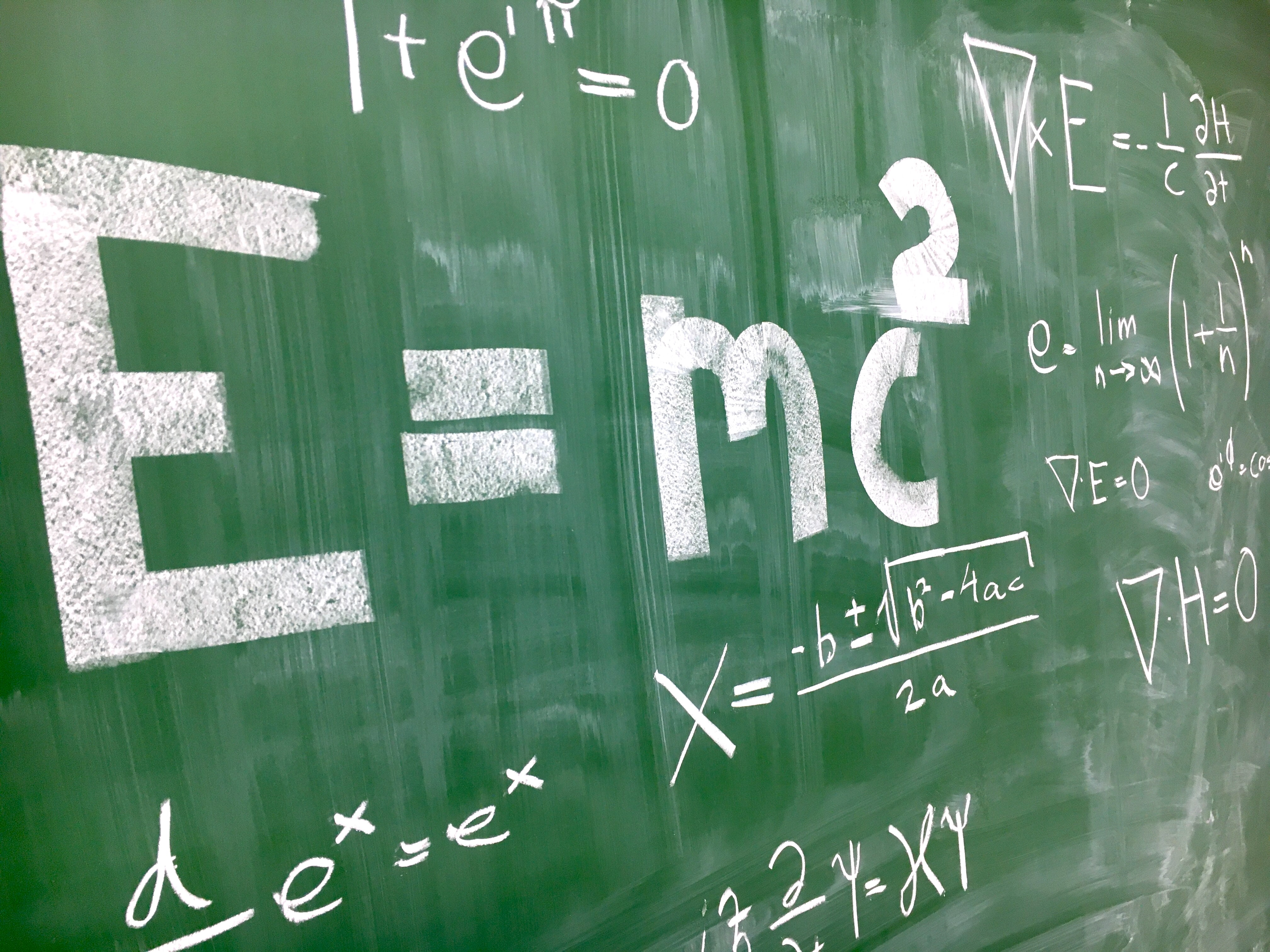# Imaginary numbers could be needed to describe reality

### By Ben Turner

#### If standard quantum theory holds up, imaginary numbers are critical.

Imaginary numbers are necessary to accurately describe reality, two new studies have suggested.

Imaginary numbers are what you get when you take the square root of a negative number, and they have long been used in the most important equations of quantum mechanics, the branch of physics that describes the world of the very small. When you add imaginary numbers and real numbers, the two form complex numbers, which enable physicists to write out quantum equations in simple terms. But whether quantum theory needs these mathematical chimeras or just uses them as convenient shortcuts has long been controversial.

In fact, even the founders of quantum mechanics themselves thought that the implications of having complex numbers in their equations was disquieting. In a letter to his friend Hendrik Lorentz, physicist Erwin Schrödinger — the first person to introduce complex numbers into quantum theory, with his quantum wave function (ψ) — wrote, "What is unpleasant here, and indeed directly to be objected to, is the use of complex numbers. Ψ is surely fundamentally a real function."Schrödinger did find ways to express his equation with only real numbers alongside an additional set of rules for how to use the equation, and later physicists have done the same with other parts of quantum theory. But in the absence of hard experimental evidence to rule upon the predictions of these "all real" equations, a question has lingered: Are imaginary numbers an optional simplification, or does trying to work without them rob quantum theory of its ability to describe reality?

Now, two studies, published Dec. 15 in the journals Nature and Physical Review Letters, have proved Schrödinger wrong. By a relatively simple experiment, they show that if quantum mechanics is correct, imaginary numbers are a necessary part of the mathematics of our universe.

"The early founders of quantum mechanics could not find any way to interpret the complex numbers appearing in the theory," lead author Marc-Olivier Renou, a theoretical physicist at the Institute of Photonic Sciences in Spain, told Live Science in an email. "Having them [complex numbers] worked very well, but there is no clear way to identify the complex numbers with an element of reality."

To test whether complex numbers were truly vital, the authors of the first study devised a twist on a classic quantum experiment known as the Bell test. The test was first proposed by physicist John Bell in 1964 as a way to prove that quantum entanglement — the weird connection between two far-apart particles that Albert Einstein objected to as "spooky action at a distance" — was required by quantum theory.In their updated version of the classic Bell test, the physicists devised an experiment in which two independent sources (which they called S and R) would be placed between three detectors (A, B and C) in an elementary quantum network. The source S would then emit two light particles, or photons — one sent to A and the other to B — in an entangled state. The source R also would emit two entangled photons, sending them to nodes B and C. If the universe were described by a standard quantum mechanics based on complex numbers, the photons that arrived at detectors A and C wouldn't need to be entangled, but in a quantum theory based on real numbers, they would.

To test this setup, the researchers of the second study performed an experiment in which they shone laser beams onto a crystal. The energy the laser gave to some of the crystals' atoms was later released as entangled photons. By looking at the states of the photons arriving at their three detectors, the researchers saw that the states of the photons arriving at detectors A and C weren't entangled, meaning their data could be described only by a quantum theory that used complex numbers.

The result makes intuitive sense; photons need to physically interact to become entangled, so those arriving at detectors A and C shouldn't be entangled if they're being produced by a different physical source. The researchers stressed, however, that their experiment only rules out theories that forgo imaginary numbers if the reigning conventions of quantum mechanics are correct. Most scientists are very confident that this is the case, but this is an important caveat nonetheless.

The result suggests that the possible ways we can describe the universe with math are actually much more constrained than we might have thought, Renou said.

"Just by observing what's coming out of some experiments, we can rule out many potential descriptions without making any assumptions [on the] reliability of the physical devices used in the experiment," Renou said. In the future, this could mean that it might just take a small number of experiments, building from first principles, for physicists to arrive at a complete quantum theory.

Beyond this, the researchers also said their experimental setup, which was a rudimentary quantum network, could be useful for outlining the principles on which a future quantum internet might operate.